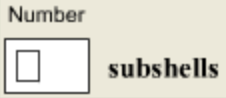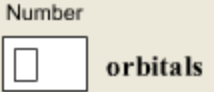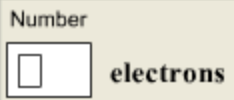In: Chemistry

# How many Subshells are in the N shell?

How many subshells are in the n = 3 shell?How many orbitals are in the n = 3 shell?What Is the maximum number of electrons in the n = 3 shell?## Solutions

##### Expert Solution

1. 3

(s,p,d)

2. 9

there are 3 subshells when n=3
which are s, p and d
the orbitals would be:
s=1
p=3
d=5
so the orbital number is 9

3. 18

Each sub-orbital can hold 2 electrons.